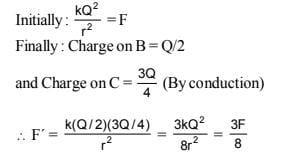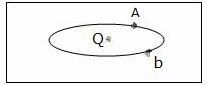NEET  >  Test: Electrostatics of Conductors

# Test: Electrostatics of Conductors

Test Description

## 10 Questions MCQ Test Physics Class 12 | Test: Electrostatics of Conductors

Test: Electrostatics of Conductors for NEET 2023 is part of Physics Class 12 preparation. The Test: Electrostatics of Conductors questions and answers have been prepared according to the NEET exam syllabus.The Test: Electrostatics of Conductors MCQs are made for NEET 2023 Exam. Find important definitions, questions, notes, meanings, examples, exercises, MCQs and online tests for Test: Electrostatics of Conductors below.
Solutions of Test: Electrostatics of Conductors questions in English are available as part of our Physics Class 12 for NEET & Test: Electrostatics of Conductors solutions in Hindi for Physics Class 12 course. Download more important topics, notes, lectures and mock test series for NEET Exam by signing up for free. Attempt Test: Electrostatics of Conductors | 10 questions in 10 minutes | Mock test for NEET preparation | Free important questions MCQ to study Physics Class 12 for NEET Exam | Download free PDF with solutions
 1 Crore+ students have signed up on EduRev. Have you?
Test: Electrostatics of Conductors - Question 1

### Two spherical conductors B and C having equal radii and carrying equal charges repel each other witha force F when kept apart at some distance. A third spherical conductor having same radius as that of B but uncharged is brought in contact with B, then brought in contact with C and finally removed awayfrom both. The new force of repulsion between B and C

Detailed Solution for Test: Electrostatics of Conductors - Question 1Test: Electrostatics of Conductors - Question 2

### As shown in the figure below, an ellipsoidal cavity is carved within a perfect conductor. A positive charge Q is placed at the centre of the cavity. If points A and b are shown on the cavity surface (see figure), then which among the following choices is correct?Detailed Solution for Test: Electrostatics of Conductors - Question 2

Electric field at A is different from field at B because, E= K/r2
we know that conductor is an equipotential surface, so potential will be the same at A and B.
As charge density, σ∝1/r​, then charge density is different at A and B.

Test: Electrostatics of Conductors - Question 3

### Electric field at the surface of a charged conductor is proportional to

Detailed Solution for Test: Electrostatics of Conductors - Question 3

Electric field at the surfaces of charged conductors is σ/ε0​.n, where n is a unit vector normal to the surface.
We clearly see that the electric field is perpendicular to surface charge density (σ).

Test: Electrostatics of Conductors - Question 4

Electrostatic field is zero_____ the conductor.

Test: Electrostatics of Conductors - Question 5

Inside a conductor, electrostatic field is:

Detailed Solution for Test: Electrostatics of Conductors - Question 5

The electric field is zero inside a conductor Because the net charge inside a conductor remains zero , the total charge of a conductor resides on its surface , as charges want to attain equilibrium so they come on surface , to minimize the  repulsion among them .As the  charge inside a conductor is zero therefore , if we apply Gauss' theorem to find the electric field inside a conductor , we find it zero .

Test: Electrostatics of Conductors - Question 6

Some charge is being given to a conductor. Then its potential:

Detailed Solution for Test: Electrostatics of Conductors - Question 6

Given that some charge is given to a conductor then the whole charge is distributed over its surface only. Inside the conductor, the electric field is zero whereas potential is the same as on the surface. Hence, throughout the conductor, potential is the same i.e, the whole conductor is equipotential.

Test: Electrostatics of Conductors - Question 7

The value of electric potential throughout the volume of a conductor is

Detailed Solution for Test: Electrostatics of Conductors - Question 7

Since the electric field inside the conductor is zero and has no tangential component on its surface, therefore, no work is done in moving a test charge within the conductor or on its surface. It means the potential difference between any two points inside or on the surface is zero. Hence, electrostatic potential is constant throughout the volume of the charged conductor and has the same value on its surface as inside it.

Test: Electrostatics of Conductors - Question 8

How does the charge densities of conductors vary on an irregularly shaped conductor?

Detailed Solution for Test: Electrostatics of Conductors - Question 8

On an irregularly shaped conductor, the surface charge density is greatest at the locations where the radius of curvature of the surface is smallest i.e, charge density is inversely proportional to radius of curvature of surface. Since the radius of curvature is least at sharp portion and maximum at flat portion, so, charge density is high at sharp portions and less at flat portions.

Test: Electrostatics of Conductors - Question 9

Two conductors having same type of charges are connected by a conducting wire. There would not be any amount of charges on them if:

Detailed Solution for Test: Electrostatics of Conductors - Question 9

When there is potential difference across the conductor, the electric field is set up. Due to this charge will flow across the conductor. But when conductors have the same potential the charge will not flow from one conductor to the other.

Test: Electrostatics of Conductors - Question 10

Consider a neutral conducting sphere. A positive point charge is placed outside the sphere. The net charge on the sphere is then,

Detailed Solution for Test: Electrostatics of Conductors - Question 10

If a charge q is placed outside than the electric field lines incident on the conducting sphere , if some charge is developed due to thee lines than opposite surface becomes oppositely charge and the total charge becomes zero

## Physics Class 12

157 videos|452 docs|213 tests
 Use Code STAYHOME200 and get INR 200 additional OFF Use Coupon Code
Information about Test: Electrostatics of Conductors Page
In this test you can find the Exam questions for Test: Electrostatics of Conductors solved & explained in the simplest way possible. Besides giving Questions and answers for Test: Electrostatics of Conductors, EduRev gives you an ample number of Online tests for practice

## Physics Class 12

157 videos|452 docs|213 tests

### How to Prepare for NEET

Read our guide to prepare for NEET which is created by Toppers & the best Teachers